Tamilnadu State Board New Syllabus Samacheer Kalvi 12th Physics Guide Pdf Chapter 5 Electromagnetic Waves Text Book Back Questions and Answers, Notes.

## Tamilnadu Samacheer Kalvi 12th Physics Solutions Chapter 5 Electromagnetic Waves

### 12th Physics Guide Electromagnetic Waves Text Book Back Questions and Answers

Part I:

Text Book Evaluation:

I. Multiple Choice Questions:

Question 1.
The dimension of $$\frac{1}{\mu_{0} \varepsilon_{0}}$$
(a) [LT-1]
(b) [L2 T-2]
(c) [L-1T]
(d) [L-2 T2]
(b) [L2 T-2]
Solution:
Dimension of µ0 = MLT-2A-2
Dimension of ε0 = M-1L-3 T4A2
∴ Dimension of = 1 µ0ε0Question 2.
If the amplitude of the magnetic field is 3 × 10-6 T, then amplitude of the electric field for a electromagnetic waves is
(a) 100 Vm-1
(b) 300 Vm-1
(c) 600 Vm-1
(d) 900 Vm-1
Ans:
(d) 900 Vm-1
Solution:
Velocity of em wave C = $$\frac{\mathrm{E}_{0}}{\mathrm{~B}_{0}}$$
E0 = C × B0 = 3 × 108 × 3 × 10-6 = 900 Vm-1

Question 3.
Which of the following electromagnetic radiation is used for viewing objects through fog
(a) Micro wave
(b) gamma rays
(c) x-rays
(d) infrared
(d) infrared
Solution:
Infrared ray can pass through mist, fog, cloud etc.,

Question 4.
Which of the following is false for electromagnetic waves
(a) transverse
(b) mechanical waves
(c) longitudinal
(d) produced by accelerating charges
(c) longitudinal
Solution:
Electromagnetic wave does not need any medium for its propagation. So it is non-mechanical, transverse wave.

Question 5.
Consider an oscillator which has a charged particle oscillating about its mean position with a frequency of 300 MHz. The wavelength of electro magnetic waves produced by this oscillator is
(a) 1 m
(b) 10 m
(c) 100 m
(d) 1000 m
(a) 1 m
Solution:
f = 300MHz = 3 × 108 HZ
Velocity of electromagnetic waveQuestion 6.
The electric and the magnetic fields, associated with an electromagnetic wave, propagating along negative x axis can be represented by
(a) $$\overrightarrow{\mathrm{E}}$$ = E0 î and B = B0
(b) $$\overrightarrow{\mathrm{E}}$$ = E0 k̂ and B = B0
(c) $$\overrightarrow{\mathrm{E}}$$ = E0 î and B = B0
(d) $$\overrightarrow{\mathrm{E}}$$ = E0 ĵ and B = B0
(d) $$\overrightarrow{\mathrm{E}}$$ = E0 ĵ and B = B0
Solution: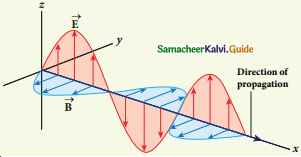Question 7.
In an electromagnetic wave in free space the rms value of the electric field is 3 Vm-1. The peak value of the magnetic field is
(a) 1.414 × 10-8 T
(b) 1.0 × 10-8 T
(c) 2.828 × 10-8 T
(d) 2.0 × 10-8 T
(a) 1.414 × 10-8 T
Solution: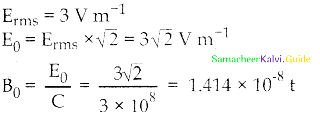Question 8.
An e.m. wave is propagating in a medium with a velocity $$\overrightarrow{\mathrm{v}}$$ = vî . The instantaneous oscillating electric field of this e.m. wave is along +y-axis, then the direction of oscillating magnetic field of the e.m. wave will be along.
(a) – y direction
(b) – x direction
(c) + z direction
(d) – z direction
(c) + z direction

Question 9.
If the magnetic monopole exists, then which of the Maxwell’s equation to be modified?
(a)(b)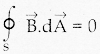(c)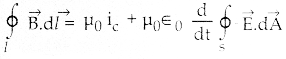(d)(b)Solution:
In this equation displacement current not taken into account. So it should be modified.

Question 10.
Fraunhofer lines are an example of ………… spectrum.
(a) line emission
(b) line absorption
(c) band emission
(d) band absorption
(b) line absorptionQuestion 11.
Which of the following is an electromagnetic wave?
(a) α – rays
(b) β – rays
(c) γ – rays
(d) all of them
(c) y – rays
Solution:
α – rays – Helium Nucleus (2He4)
β – rays – Electron (e-1)
γ – rays – Quantum of electromagnetic energy

Question 12.
Which one of them is used to produce a propagating electromagnetic wave?
(a) an accelerating charge
(b) a charge moving with constant velocity
(c) a stationary charge
(d) an uncharged particle
(a) an accelerating charge
Solution:
By changing the electric field at a point alone can produce a change in the magnetic field at that point. Hence, an electromagnetic wave can propagate.

(b) a charge moving at constant velocity has no acceleration. So, the electric field will not charge

Question 13.
Let E = E0 sin [106x – ωt] be the electric field of plane electromagnetic wave, the value of ω is
(a) 0.3 × 10-4 rad s-1
(b) 3 × 10-14 rad s-1
(c) 0.3 × 1014 rad s-1
(d) 3 × 1014 rad s-1
(d) 3 × 1014 rad s-1
Solution:Question 14.
Which of the following is NOT true for electromagnetic waves?
(a) it transports energy
(b) it transports momentum
(c) it transports angular momentum
(d) in a vacuum, it travels at different speeds which depend on its frequency
(d) in a vacuum, it travels at different speeds which depend on its frequency
Solution:
Reason:
All electromagnetic waves with different frequencies travel with the same velocity 3 × 108 ms-1 in a vacuum. But in medium depending upon their wavelength the speed varies. Cm α $$\frac{1}{\lambda}$$

Question 15.
The electric and magnetic fields of an electromagnetic wave are
(a) in phase and perpendicular to each other
(b) out of phase and not perpendicular to each other
(c) in phase and not perpendicular to each other
(d) out of phase and perpendicular to each other
(a) in phase and perpendicular to each other
Solution:
In electromagnetic wave Ey = E0 sin (ky – ωt) Bz = B0 sin (kz – ωt) phase difference between electric and magnetic components in electromagnetic wave is zero ∆Φ = 0Question 1.
What is displacement current?
The displacement current can be defined as the current which comes into play in the region in which the electric field and the electric flux are changing with time.

Question 2.
What are electromagnetic waves?
1. Electromagnetic waves are non-mechanical waves that move with speed equals to the speed of light (in a vacuum).
2. It is a transverse wave.

Question 3.
Write down the integral form of modified Ampere’s circuital law.
This law relates the magnetic field around any closed path to the conduction current and displacement current through that path.Question 4.
Write notes an Gauss’ law in magnetism.
The surface integral of magnetic field over a closed surface is zero mathematicaly,
$$\oint$$$$\vec{B}$$.d$$\vec{A}$$ = 0 where B is the magnetic field.

Question 5.
Give two uses for each of the following.
(ii) Microwaves and

1. To produce dehydrated fruits
2. TV remote as a signal comer.

(ii) Microwaves:

2. Microwave oven for cooking

1. To destroy bacteria
2. To burglar alarm.Question 6.
What are Fraunhofer lines? How are they useful in the identification elements present in the sun?
When the spectrum obtained from the Sun is examined, it consists of a large number of dark lines (line absorption spectrum). These dark lines in the solar spectrum are known as Fraunhofer lines.

Question 7.
Write notes on Ampere – Maxwell law.
Maxwell modified Ampere’s law aswhere the total current enclosed by the surface becomes the sum of conduction current and displacement current. The equation (1) is known as Ampere – Maxwell law.

Question 8.
Why are e.m waves non-mechanical?
Electromagnetic waves are produced by an accelerated charge and do not require any medium for propagation. So electromagnetic wave is a non-mechanical wave.Question 1.
White down Maxwell equations in integral form.
1. Electrodynamics can be summarized into four basic equations, known as Maxwell’s Equations.
2. First equation is Gauss Law, it relates net electric flux and net charge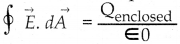3. Second equation has no name but can be called Gauss Law in magnetism. It states that the surface integral of the magnetic field over a closed surface is zero.
$$\vec{B}$$ . d$$\vec{A}$$ = 0

4. Third equation is Faraday’s Laws of electromagnetic induction.
$$\oint$$ $$\vec{E}$$ . d$$\vec{l}$$ = – $$\frac{d}{d t}$$ ΦB

5. The line integral of the electric field ground any closed path is equal to the rate of change of magnetic flux through the closed path bounded by the surface.

6. Fourth Equation is modified Ampere’s Circuital Law known as Ampere – Maxwell’s Law7. This law relates the magnetic field around any closed path to the conduction current and displacement current through the path. These four equations are known as Maxwell’s Equation in electrodynamics.

Question 2.
Write short notes on
(a) Microwave
(b) X – Ray
(d) Visible Spectrum
Microwaves:
It is produced by electromagnetic oscillators in electric circuits. The wavelength range is 1 x 10-3 m to 3 x 10-1 m and frequency range is 3 x 1011 Hz to 1 x 109 Hz. It obeys reflection and polarization. It is used in radar system for aircraft navigation, speed of the vehicle, microwave oven for cooking and very long-distance wireless communication through satellites.

X-rays:
It is produced when there is a sudden deceleration of high speed electrons at high- atomic number target, and also by electronic transitions among the innermost orbits of atoms. The wavelength range 10-13 m to 10-8 m and frequency range are 3 x 1021 Hz to 1 x 1016 Hz. X-rays have more penetrating power than ultraviolet radiation.

X-rays are used extensively in studying structures of inner atomic electron shells and crystal structures. It is used in detecting fractures, diseased organs, formation of bones and stones, observing the progress of healing bones. Further, in a finished metal product, it is used to detect faults, cracks, flaws and holes.

It is produced by oscillators in electric circuits. The wavelength range is 1 x 10-1 m to 1 x 104 m and the frequency range is 3 x 109 Hz to 3 x 104 Hz. It obeys reflection and diffraction. It is used in radio and television communication systems and also in cellular phones to transmit voice communication in the ultrahigh-frequency band.

Visible light:
It is produced by incandescent bodies and also it is radiated by excited atoms in gases. The wavelength range is 4 x 10-7 m to 7 x 10-7 m and the frequency range is 7 x 1014 Hz to 4 x 1014Hz. It obeys the laws of reflection, refraction, interference, diffraction, polarization, photoelectric effect, and photographic action. It can be used to study the structure of molecules, the arrangement of electrons in external shells of atoms, and the sensation of our eyes.Question 3.
Discuss the Hertz experiment.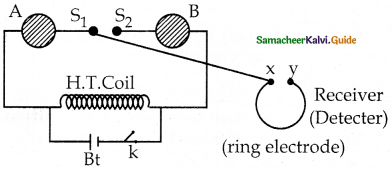The existence of electromagnetic waves was experimentally confirmed by Hertz in 1888.

Construction:

1. It consists of two metal electrodes (S1, S2) which are made of small spherical metals.
2. These are connected to large spheres (A, B) and the ends of them are connected to an induction coil with a very large number of turns (H.T. Coil)

Working:

1. The induction coil produces a very high electromotive force (emf)
2. Since the coil is maintained at very high potential are between the electrodes (S1, S2) get ionized and spark is produced.
3. The gap between the ring type electrode kept at a distance also gets spark.
4. This implies that energy is transmitted from an electrode (S1, S2) to the receiver (x, y)
5. If the receiver (x, y) is rotated by 90° no spark is observed by the receiver.
6. This confirms that electromagnetic waves are transverse.
7. Hertz produced radio wave which also travels with the velocity of light (3 × 108 ms-1).

Question 4.
Explain the Maxwell’s modification Ampere’s circuital law.
Ampere’s circuital law is $$\oint$$$$\vec{B}$$.d$$\vec{s}$$ = µ0I
Modification by J.C. Maxwell on Ampere’s circuital law1. Due to external source applied between the plates, the increasing current flowing through the capacitor produce an increasing electric field between the plates.

2. This change in the electric field between the capacitor plate produce a current between the plates.

3. The time varying electric flux between the plate of the capacitor produce a current known as displacement current.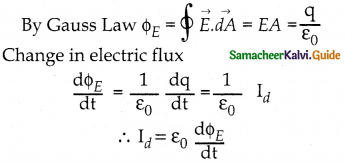Id is the displacement current.

4. The displacement current is defined as the current which comes into play in the region in which the electric field and the electric flux are changing with time.

5. So, Maxwell modified Ampere’s Law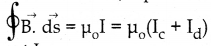Total current I = conduction current Ic + displacement current IdQuestion 5.
Explain the importance of Maxwell’s correction.
1. Ampere’s law says that only an electric current can produce a magnetic field. If Ampere’s law alone is true, there will not be anv radiation.

2. Maxwell’s correction term $$\left(\mu_{0} \varepsilon_{0} \frac{\mathrm{d} \phi_{E}}{\mathrm{dt}}\right)$$

3. In ampere’s law ensures that time varying electric field or displacement current can also produce magnetic field.

4. Though conduction current is zero in an empty space displacement current does exist. So Maxwell equation becomes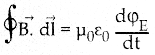5. Faraday’s law this time-varying magnetic field produces again time-varying electric field and so on.

6. The coupled time varying electric and magnetic fields travel through empty space with the speed of light and is called electromagnetic wave.

7. Even though Maxwell initially started with a purely symmetry argument his correction term explains one of the important aspects of the universe namely the existence of electro magnetic waves.

Question 6.
Write down the properties of electromagnetic waves.
1. Electromagnetic waves are produced bv any accelerated charges.

2. Do not require any medium for propagation. They are non-mechanical wave.

3. Electric field vector, magnetic field vector and direction of propagation of electromagnetic waves are mutually perpendicular to each other. Hence they are transverse in nature.

4. They travel in free space or vacuum with a velocity C = 3 × 108 ms-1 and it is given by the expression C = $$\frac{1}{\sqrt{\mu_{0} \varepsilon_{o}}}$$

5. In medium electromagnetic wave travel with velocity less than speed in free space or vacuum.
velocity in medium V = $$\frac{C}{\mu}=\frac{1}{\sqrt{\varepsilon_{\mathrm{r}} \mu_{\mathrm{r}}}}$$

6. They are not deflected by electric and magnetic fields.

7. They can undergo interference, diffraction and polarisation.

8. The energy density of electromagnetic wave is
U = εoE2 = $$\frac{1}{\mu_{\mathrm{o}}}$$ B2

9. The average energy’ density’ of electromagnetic wave (U) = $$\frac{1}{2} \varepsilon_{\mathrm{o}} \mathrm{E}^{2}=\frac{1}{2} \frac{1}{\mathrm{\mu}_{\mathrm{O}}} \mathrm{B}^{2}$$

10. They carry’ energy’ and linear momentum which is equal to11. If electromagnetic waves of energy’ U in completely absorbed by a surface, the momentum imparted on the surface is
P = $$\frac{\mathrm{U}}{\mathrm{C}}$$

12. If an electromagnetic wave of energy U is completely reflected by the surface, momentum delivered to the surface is
Δp = $$\frac{2 \mathrm{U}}{\mathrm{C}}$$

13. Rate of flow of energy crossing per unit area is known as pointing vector for electromagnetic wave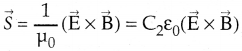14. Along with energy, linear momentum of the electromagnetic wave also has angular momentum.

Question 7.
Discuss the source of electromagnetic waves.
The source of electromagnetic wave is accelerating electric charges.

Explanation:
1. Stationary charges produce only electric field.
2. All oscillatory motions are accelerating motion.
3. If a charge is accelerated along x-axis, it produce a change in electric field along y-axis Ey = E0 sin (kz – ωt)
4. A linked magnetic field produced mutually perpendicular to electric field direction, so it point along x-axis, Bx = B0 sin (kz – ωt)
5. Now both the electric field vectors and magnetic field vectors have the property of a wave and propagate along z-axis with the velocity of light.
6. n free space or in vacuum, the ratio between E0 and B0 is equal to the speed of

Propagation of an Electromagnetic waveOscillating charges – sources of electromagnetic waves

electromagnetic wave C = $$\frac{E_{0}}{B_{0}}$$
But in medium velocity v = $$\frac{E_{0}}{B_{0}}$$ < C
7. Energy of electromagnetic waves comes from the energy of oscillating charge.Question 8.
Explain the types of emission spectrum.
1. Emission spectra:
When the spectrum of self luminous source is taken, we get emission spectrum. Each source has its own characteristic emission spectrum.
Types:
1. Continuous Emission Spectra
2. Line emission spectra
3. Band emission spectra

1. Continuous Emission Spectra:
It consist of wavelength containing all the visible colours ranging from violet to red. Example: Spectrum from carbon arc, incandescent solids, liquids.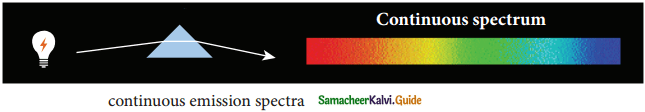2. Line Emission Spectra:
1. Line spectra are sharp lines of definite wave length or frequencies.
2. Light from hot gas is allowed to pass through a prism, we get line spectrum (or) discontinuous spectrum.
3. Line spectra arises due to excited atoms of element.
4. They are the characteristics of the element. Example: Spectra of atomic hydrogen, helium etc.3. Band Emission Spectra:
1. Band spectrum consists of several numbers of very closely spaced spectral lines which overlapped together forming specific bands which are separated by dark spaces known as band spectra.

2. This spectrum has a sharp edge at one end and fades out at the other end.

3. This spectrum arises when molecules are excited so, they are the characteristics of molecules. Used to study the structure of molecules.
Example: Spectra of hydrogen gas, ammonia gas in the discharge tube.

Question 9.
Explain the types of the absorption spectrum.
Absorption Spectra: When light is allowed to pass through an absorbing substance then the spectrum obtained is known as absorption spectra. This spectrum is the characteristic of absorbing substances.

Types:

1. Continuous absorption spectrum
2. Line absorption spectrum
3. Band absorption spectrum

1. Continuous absorption spectrum:
When light is passed through a medium then if it is dispersed by a prism, we get continuous absorption spectrum.
Example: When white light passes through a blue glass plate, it absorbs everything except blue.

2. Line absorption spectrum:
When white light from the incandescent lamp is passed through cold gas, the spectrum obtained through the dispersion due to prism is line absorption spectrum.
Example:
When light from carbon arc made to pass through sodium vapour lamp; a continuous spectrum of carbon arc with two dark line in the yellow region is obtained.3. Band absorption spectrum:
When white light is pass through iodine vapour or diluted solution of blood or chlorophyll or through certain solutions of organic and inorganic compounds dark bands as the continuous bright background is obtained.IV. Numerical Problems:

Question 1.
Consider a parallel plate capacitor whose plates are closely spaced. Let R be the radius of the plates and the current in the wire connected to the plates is 5 A, calculate the displacement current through the surface passing between the plates by directly calculating the rate of change of flux of electric field through the surface.
Id = Ic = 5A
Id = $$\frac{\mathrm{d} \phi}{\mathrm{dt}}$$
Id = 5 A

Question 2.
A transmitter consists of an LC circuit with an inductance of 1 µH and capacitance of 1 µF. What is the wavelength of the electromagnetic waves it emits?
18.84 × 10-6m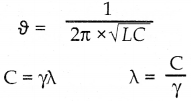= C × 2π × √LC
= 3 × 108 × 6.28 × 10-6 × 10-6
= 3 × 108 × 6.28 × 10-6
= 18.86 × 102 m

Question 3.
A pulse of light of duration 10-6 s is absorbed completely by a small object initially at rest. If the power of the pulse is 60 × 10-3 W, calculate the final momentum of the object.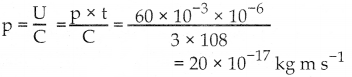Question 4.
Let an electromagnetic wave propagation along the x-direction, the magnetic field oscillates at a frequency of 1010 Hz and has an amplitude of 10-5 T, acting along the y-direction. Then, compute the wavelength of the wave. Also, write down the expression for the electric field in this case.
Given Data:
f = 1010 Hz
B0 = 10-5T
1. Wavelength λ = ?
2. Expression for electric field Ex = ?
The velocity of Electromagnetic wave in free space
C = $$\frac{\mathrm{E}_{0}}{\mathrm{~B}_{0}}$$
∴ E0 = C × B0 = 3 × 108 × 10-5
E0 = 3 × 103 NC-1
Wavelength λ = $$\frac{C}{f}=\frac{3 \times 10^{8}}{10^{10}}$$ = 3 × 10-2 m

(i) wavelength of electromagnetic wave λ = 3 × 10-2 m

(ii) Expression for electric field:
Ez = E0 sin (kz – ωt)
wave number k = $$\frac{2 \pi}{\lambda}$$ = 2π/3 × 10-2 = 0.66π × 102 A
k = 66π m-1 = 2.09 × 102
Angular frequency ω = 2πf = 2π × 1010 rad s-1
= 3 × 103 sin (2.09 × 102x – 6.28 × 1010t)Question 5.
If the relative permeability and relative permittivity of a medium is 1.0 and 2.25, respectively. Find the speed of the electromagnetic wave in this medium.
Given Data:
µr = 1.0, εr = 2.25
Velocity of electromagnetic wave in medium
v = ?
The velocity of an electromagnetic wave in a medium
V = $$\frac{C}{\mu}$$
Refractive index µ = $$\sqrt{\varepsilon r \mu r}$$Part II:

I. Matching Type Questions:

Question a.(1) A → b, B → c, C → d, D → a
(2) A → d, B → a, C → b, D → c
(3) A → c, B → d, C → b, D → a
(4) A → c, B → a, C → d, D → b
(3) A → c, B → d, C → b, D → a

Question b.(1) A → d, B → C, C → a, D → b
(2) A → c, B → a, C → d, D → b
(3) A → b, B → C, C → d, D → a
(4) A → d, B → a, C → b, D → C
(1) A → d, B → C, C → a, D → bQuestion c.

 (A)  Microwave a. Night vision photography (B) Ultraviolet radiation b. Crystal structure (C) X-rays c. Molecular structure (D) Infrared radiation d. Aircraft navigation

(1) A → c, B → d, C → b, D → a
(2) A → b, B → c, C → d, D → a
(3) A → d, B →c, C → b, D → a
(4) A → d, B → a, C → b, D → c
Ans:
(3) A → d, B →c, C → b, D → a

Question d.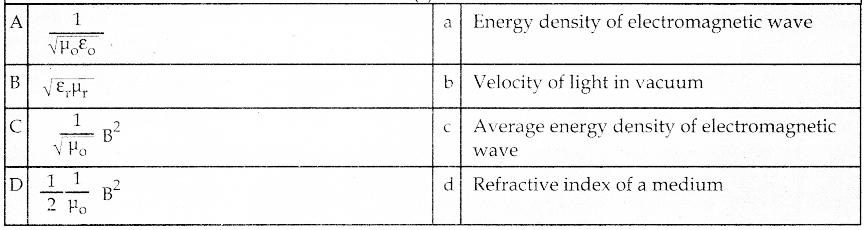(1) A → d, B → a, C → b, D → c
(2) A → c, B → a, C → d, D → b
(3) A → d, B → c, C → a, D → b
(4) A → b, B → d, C → a, D → c
(4) A → b, B → d, C → a, D → c

Question e.

 (A) Carbon arc a. Line emission spectrum (B) Spectra of atomic helium b. Band emission spectra (C) Molecular structure c. Line absorption spectra (D) Spectrum obtained from sun d. Continuous emission spectrum

(1) A → d, B → a, C → b, D → c
(2) A → c, B → a, C → d, D → b
(3) A → c, B → d, C → b, D → a
(4) A → b, B → d, C → a, D → c
(1) A → d, B → a, C → b, D → cII. Fill in the blanks:

Question 1.
Electromagnetic wave are _________ in nature.
Transverse

Question 2.
The phase difference between electric and magnetic field vectors in an electromagnetic wave _________.
Zero

Question 3.
The angle between electric and magnetic component in an electromagnetic wave is _________.
$$\pi / 2$$ (or) 90°

Question 4.
Linear momentum of electromagnetic wave is _________.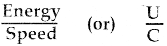Question 5.
The rate of flow of energy crossing in unit area is known as _________.
Pointing vectorQuestion 6.
The expression for intensity of electromagnetic wave is equal to _________.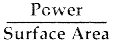Question 7.
The electromagnetic wave from the sun which is absorbed by atmospheric ozone is _________.
Ultraviolet rays

Question 8.
The electromagnetic wave produced by Hertz is _________.

Question 9.
When white light passing through chlorophyll it gives _________.
Band absorption spectrum

Question 10.
The wavelength range of visible light is _________ to _________.
4 × 10-7m to 7 × 10-7 mIII. Choose the Odd One Out:

Question 1.
a) Velocity of light in vacuum
b) 3 × 108 ms-1
c) $$\frac{1}{\sqrt{\mu_{0}} \varepsilon_{0}}$$
d) $$\sqrt{\mu_{\mathrm{r}} \varepsilon_{\mathrm{r}}}$$
d) $$\sqrt{\mu_{\mathrm{r}} \varepsilon_{\mathrm{r}}}$$

Question 2.
a) Fraunhofer
b) J.C. Maxwell
c) Hertz
d) Michael
a) Fraunhofer

Question 3.
a) x-ray
b) Gamma rays
c) Microwave
d) Ultraviolet ray
c) Microwave

Question 4.
a) Ampere – Maxwell law

b) $$\oint \overrightarrow{\mathrm{E}} \cdot \overrightarrow{\mathrm{d} \mathrm{t}}=\frac{\mathrm{d} \phi_{\mathrm{B}}}{\mathrm{dt}}$$

c) $$\oint \overrightarrow{\mathrm{B}} \cdot \overrightarrow{\mathrm{d} s}=\mu_{0}\left(\mathrm{I}_{\mathrm{c}}+\mathrm{I}_{\mathrm{d}}\right)$$

d) $$\oint \overrightarrow{B .} \overrightarrow{d l}$$ = $$\mu_{0} \quad I_{0}+\mu_{0} \varepsilon_{0} \frac{d}{d t} \int_{S} \vec{E} \cdot d \vec{A}$$
b) $$\oint \overrightarrow{\mathrm{E}} \cdot \overrightarrow{\mathrm{d} \mathrm{t}}=\frac{\mathrm{d} \phi_{\mathrm{B}}}{\mathrm{dt}}$$

Question 5.
a) $$\varepsilon_{0}=\frac{\mathrm{d} \phi_{\mathrm{B}}}{\mathrm{dt}}$$
b) Displacement current
c) μ0I0
d) $$\varepsilon_{0} \frac{\mathrm{d}}{\mathrm{dt}} \int_{\mathrm{S}} \overrightarrow{\mathrm{E} \cdot \mathrm{d}} \overrightarrow{\mathrm{A}}$$
c) μ0I0IV. Choose the Incorrect Pair:

Question 1.
a) Electromagnetic wave – Transverse
b) The ratio between the amplitude of the electric wave and magnetic components is an electromagnetic wave – Speed of electromagnetic wave
d) Momentum imparted by electromagnetic p = $$\frac{\mathrm{U}}{\mathrm{C}}$$

Question 2.
a) Atomic spectrum – pure line spectrum
b) Solar spectrum – line spectrum
c) Molecules – Band Spectrum
d) Carbon arc – Continuous emission spectrum
b) Solar spectrum is the line absorption spectrum

Question 3.
a) Refractive index of a medium – μ = $$\sqrt{\varepsilon_{\mathrm{r}} \mathrm{H}_{\mathrm{r}}}$$
b) Pointing vector – $$\vec{S}=C^{2} \varepsilon_{0}(\vec{B} \times \vec{E})$$
c) To detect faults, cracks, flaws, and holes – X-rays
d) Fraunhofer lines – Sun’s atmosphere
b) Pointing vector – $$\vec{S}=C^{2} \varepsilon_{0}(\vec{B} \times \vec{E})$$

Question 4.
a) Net electric flux and net charge – Gauss law
b) Electric field and magnetic flux – Faraday’s law
c) Magnetic field around any closed path to the conduction current – Maxwell’s law
d) Magnetic field around any closed path to the conduction current and displacement current through that path – Ampere – Maxwell’s law
c) Magnetic field around any closed path to the conduction currentV. Choose the correct pair:

Question 1.
a) Wavelength range of radio wave – 3 × 109 m to 3 × 104 m
b) Wavelength range of ultraviolet radiation – 5 × 1017 m to 7 × 1014 m
c) Wavelength range of Gamma radiation – 10-14 m to 1 × 10-10 m
d) Wavelength range of Infrared radiation – 4 × 1014 to 6 × 1010 m
c) Wavelength range of Gamma radiation – 10-14 cm to 1 × 10-10 m

Question 2.
a) Incandescent solids – Band absorption spectra
b) Spectrum from carbon arc – Line spectrum
c) Spectra of atomic hydrogen – Line absorption spectrum
d) the characteristics of substance – Absorption spectrum
d) the characteristics of substance – Absorption spectrum

Question 3.
a) Maxwell’s prediction on the electromagnetic wave was verified by – Ampere
b) Rate of change in the magnetic field produces electric field – Gauss
c) Total electric flux is equal to 1 ε/0 times net charge enclosed by the surface – Gauss law
d) An example of mechanical wave – Electromagnetic waves
c) Total electric flux is equal to 1 ε/o times net charge enclosed by the surface – Gauss law

Question 4.
a) Velocity of x-ray in vacuum is – 3 × 108 ms-1
b) Velocity of x-ray in medium – 3 × 108 ms-1
c) Electromagnetic waves – Deflected by both electric and magnetic field
d) The electric component of electromagnetic wave – Ez = E0 Sin (kz – ωt) ms-1
a) Velocity of x-ray in vacuum is – 3 × 108 ms-1VI. Assertion and Reason:

Question 1.
Assertion (A):
The velocity of electromagnetic waves in a medium is always less than the velocity of electromagnetic wave in free space (or) vacuum
Reason (R):
The wavelength of the electromagnetic wave decreases when traveling into a denser medium but frequency does not change
a) A is correct R is wrong
b) A is wrong R is correct
c) A and R are correct
d) A and R are wrong
c) A and R are correct

Question 2.
Assertion (A):
Refractive index of air is $$\mu=\sqrt{\mu_{0}}$$
Reason (R):
Since the dielectric constant of air equal to one (εr = 1 for air). The Refractive index of air $$\mu=\sqrt{\mu_{r}}$$
a) A is correct R is wrong
b) A is wrong R is correct
c) A and R are correct
d) A and R are wrong
b) A is wrong R is correct

Question 3.
Assertion (A):
$$\oint \overrightarrow{\mathrm{E}} \cdot \overrightarrow{\mathrm{d} A}=\frac{\mathrm{Q}_{\text {enclosed }}}{\epsilon_{0}}$$
$$\oint_{\vec{B}} \cdot \overrightarrow{\mathrm{d} A}$$ (Gauss law in electrostatics)
Reason (R):
$$\oint \overrightarrow{\mathrm{E}} \cdot \overrightarrow{\mathrm{d} A}=\frac{\mathrm{Q}_{\text {enclosed }}}{\epsilon_{0}}$$
$$\oint_{\vec{B}} \cdot \overrightarrow{\mathrm{d} A}$$ since isolated charges cannot exist since isolated magnetic monopole exist.
a) A is correct R is wrong
b) A is wrong R is correct
c) A and R are correct
d) Aand Rare wrong
a) A is correct R is wrong

Question 4.
Assertion (A):
All medium other than air has relative permittivity less than one
Reason (R):
Electromagnetic wave travels faster in a medium than in air
a) A is correct R is wrong
b) A is wrong R is correct
c) A and R are correct
d) A and R are wrong
d) A and R are wrongVII. Choose the correct statement:

Question 1.
a) Maxwell’s law of induction states that Time-varying electric field produces a magnetic field
b) Faraday’s law of induction states that Time-varying electric field produces a magnetic field
c) Hertz experimentally proved accelerated charges produce an only magnetic field
d) Ampere’s law states that total flux over any surface enclosing a charge q is always zero.
a) Maxwell’s law of induction states that Time-varying electric field produces a magnetic field

Question 2.
a) Electromagnetic waves need a medium for its propagation
b) Electromagnetic waves are mostly transverse, it can also longitudinal wave
c) Electromagnetic waves are produced by any accelerated charges
d) The ratio between electric energy and magnetic energy in an electromagnetic wave is 2:1
c) Electromagnetic waves are produced by any accelerated charges

Question 3.
a) Electromagnetic waves have only linear momentum no angular momentum.
b) The energy density of the electromagnetic wave is u = $$\frac{\beta^{2}}{2 \mu_{0}}$$
c) The average energy density of electromagnetic wave is u = $$\frac{\beta^{2}}{\mu_{0}}$$
d) If the electromagnetic wave incident on a surface, the momentum imparted as the surface is p = $$\frac{\mathrm{u}}{\mathrm{c}}$$
d) If the electromagnetic wave incident on a surface, the momentum imparted as the surface is p = $$\frac{\mathrm{u}}{\mathrm{c}}$$

Question 4.
a) Cellular phones used infrared radiation.
b) Molecular spectrum is sharp at one end and fades out at the other end.
c) When light from the incandescent lamp passed through cold gas, the spectrum obtained is the Band absorption spectrum.
d) Fraunhofer lines help to identify the elements in the earth’s atmosphere.
b) Molecular spectrum is sharp at one end and fades out at the other end.VIII. Choose the Incorrect Statement:

Question 1.
a) According to Faraday change in the magnetic field at a point with respect to time produces an electric field at that point
b) Amperes law is the line integral of the magnetic field $$\vec{B}$$ around any closed loop is equal to iiü times the net current I threading through the area enclosed by the loop.
c) Total current enclosed by a surface is the sum of conduction current and displacement current
I = Ic + Id
d) When a constant current is applied between the plates of capacitor Ic = 0 and hence I = Id
d) When a constant current is applied between the plates of capacitor Ic = 0 and hence I = Id

Question 2.
a) Velocity of an electromagnetic wave in a medium of refractive index 1.5 is 2.25 × 108 ms-1
b) If the velocity of the electromagnetic wave is 2 × 108 ms-1 the refractive index of the medium is 2
c) If the velocity of light is 2.25 x 108 ms-1 the medium must have a refractive index of 1.33
d) If the refractive index of a medium increases the velocity of electromagnetic wave decreases.
c) If the velocity of light is 2.25 x 108 ms-1 the medium must have a refractive index of 1.33

Question 3.
a) Intensity of electromagnetic wave I = $$\frac{\text { Energy }(\mathrm{U})}{\text { Area }(\mathrm{A}) \times \text { Time }(\mathrm{T})}$$
b) Intensity of electromagnetic wave I = $$\frac{\text { Power }(\mathrm{P})}{\text { Surface Area }(\mathrm{A})}$$
c) Energy density in an electric field is $$\frac{1}{2}$$ ε0 E2
d) Energy density in a magnetic field is $$\frac{1}{2}$$ µ0 B2
d) Energy density in a magnetic field is $$\frac{1}{2}$$ µ0 B2

Question 4.
a) Electromagnetic waves carry not only energy and momentum but also angular momentum in
b) The dark line in the solar spectrum are known as Fraunhofer lines
c) Solar spectrum is the best example for line absorption spectrum
d) The instantaneous magnitude of the electric and magnetic field vectors in an electromagnetic wave are related by
B = Ec
d) The instantaneous magnitude of the electric and magnetic field vectors in an electromagnetic wave are related by
B = EcQuestion 1.
The speed of electromagnetic waves in vacuum is given by
(a) μ0ε0
(b) $$\sqrt { { \mu }_{ 0 }{ \varepsilon }_{ 0 } }$$
(c) $$\frac { 1 }{ { \mu }_{ 0 }{ \varepsilon }_{ 0 } }$$
(d) $$\frac { 1 }{ \sqrt { { \mu }_{ 0 }{ \varepsilon }_{ 0 } } }$$
(d) $$\frac { 1 }{ \sqrt { { \mu }_{ 0 }{ \varepsilon }_{ 0 } } }$$.

Question 2.
Electromagnetic waves are
a) Neither longitudinal nor transverse
b) Longitudinal
c) transverse
d) both longitudinal and transverse
c) transverse

Question 3.
If E and B be the electric and magnetic field vectors of an electromagnetic wave, then the propagation of the wave is along the direction of
(a) E
(b) B
(c) E x B
(d) Bx E
(c) E x B.

Question 4.
To produce a displacement of 8.854 mA between the parallel plate capacitor in 0.2 μs, the change in electric flux must be
a) 200 Wb
b) 20 Wb
c) 2 Wb
d) 0.2 Wb
a) 200 Wb
Solution: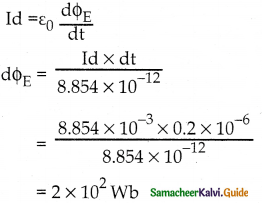Question 5.
Maxwell’s modified Ampere’s Law is
a) $$\oint \overrightarrow{\mathrm{E}} \cdot \overrightarrow{\mathrm{d} s}=\mu_{0}\left(\mathrm{I}_{\mathrm{c}}+\mathrm{I}_{\mathrm{d}}\right)$$

b) $$\oint \overrightarrow{\mathrm{E}} \cdot \overrightarrow{\mathrm{d} s}=\mu_{0} \mathrm{I}_{\mathrm{c}}$$

c) $$\oint \overrightarrow{\mathrm{B}} \cdot \overrightarrow{\mathrm{d} s}=\mu_{0} \mathrm{I}_{\mathrm{c}}$$

d) $$\oint \overrightarrow{\mathrm{B}} \cdot \overrightarrow{\mathrm{d} s}=\mu_{0}\left(\mathrm{I}_{\mathrm{c}}+\mathrm{I}_{\mathrm{d}}\right)$$
d) $$\oint \overrightarrow{\mathrm{B}} \cdot \overrightarrow{\mathrm{d} s}=\mu_{0}\left(\mathrm{I}_{\mathrm{c}}+\mathrm{I}_{\mathrm{d}}\right)$$Question 6.
Hertz produced an electromagnetic wave of frequency 5 × 107 Hz means, the wavelength of
the wave is
a) 150 m
b) 15 m
c) 6 m
d) 60 m
c) 6 m
Solution:
λ = $$\frac{C}{\gamma}=\frac{3 \times 10^{8}}{5 \times 10^{7}}=\frac{30}{5}$$ = 6 m

Question 7.
In Hertz experiment, the energy of the electromagnetic wave is
a) Kinetic energy of the oscillating charge
b) Potential energy of the charge
c) Electric energy between plates
d) Magnetic energy of the connecting wires
a) Kinetic energy of the oscillating charge

Question 8.
A medium has a refractive index of 1.5 with relative permittivity of 2 has a relative magnetic permeability
a) 11.25
b) 112.5
c) 1.125
d) 2.125
c) 1.125
Solution: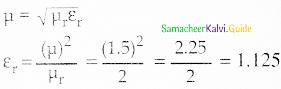Question 9.
The electromagnetic radiation most prevalent in the atmosphere is
(a) Visible light
(b) Infrared
(c) UV
(b) Infrared.

Question 10.
An electromagnetic wave of energy U is completely transferred to a surface, the momentum imparted on the surface is
a) U
b) Uc
c) U/c
d) c/U
c) U/cQuestion 11.
The average energy density of an electromagnetic wave of magnetic field 4π × 10-7 T is
a) 2π × 10-7 Jm-3
b) 4π × 10-7 Jm-3
c) 19.878 × 10-6 Jm-3
d) 6.626 × 10-7 Jm-3
a) 2π × 10-7 Jm-3
Solution: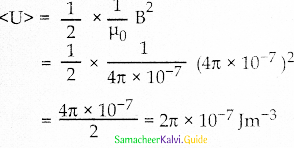Question 12.
The greenhouse effect is caused by
(a) UV rays
(b) X-rays
(c) Gamma rays
(d) IR rays
(d) IR rays.

Question 13.
The velocity of electromagnetic wave in free space or vacuum is
a) 3 × 10-8 ms-1
b) $$\sqrt{\mu_{\mathrm{o}} \varepsilon_{\mathrm{o}}}$$ ms-1
c) $$\frac{1}{\mu_{r} \varepsilon_{r}}$$ ms-1
d) $$\left(\varepsilon_{0} \mu_{0}\right)^{-1 / 2}$$ ms-1
d) $$\left(\varepsilon_{0} \mu_{0}\right)^{-1 / 2}$$ ms-1

Question 14.
The velocity of an electromagnetic wave through a medium which a permeability in free space 4π × 10-7 Hm-1 and permittivity in free space of 8.854 × 10-12 C2N-1m2 is
a) 2 × 108 ms-1
b) 3 × 108 ms-1
c) 2.25 × 108 ms-1
d) 1.5 × 108 ms-1
b) 3 × 108 ms-1
Solution: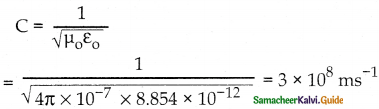Question 15.
The peak value of an electric field in an electromagnetic wave is 3√2 Vm-1. The peak value of the magnetic field is
a) 1.414 × 10-8 T
b) 1.0 × 10-8 T
c) 2.828 × 10-8 T
d) 2.0 × 10-8 T
a) 1.414 × 10-8 T
Solution: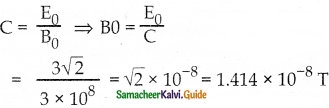Question 16.
Which of the following is not an electromagnetic wave
a) x-ray
b) u-v ray
c) β-ray
d) γ-ray
c) β-ray

Question 17.
The ratio of amplitude of magnetic field to the amplitude of electric field for an electromagnetic wave propagating in vacuum is equal to
(a) the speed of light in vacuum
(b) reciprocal of the speed of light in vacuum
(c) the ratio of magnetic permeability to electric susceptibility in vacuum
(d) unity
(b) reciprocal of the speed of light in vacuum.

Question 18.
The current in the electric circuit which arises due to the flow of electrons in the connecting wires of the circuit in a defined closed path is called
a) alternating current
b) direct current
c) conduction current
d) displacement current
c) conduction current

Question 19.
The conduction current is the same as the displacement current when the source is
a) ac only
b) dc only
c) either ac or dc
d) neither dc nor ac
c) either ac or dc

Question 20.
If a variable frequency ac source connected to a capacitor then with a decrease in frequency, the displacement current will
a) increase
b) decrease
c) remains constant
d) first decrease then increase
b) decrease
Solution:
Current through capacitor,
I = $$\frac{E}{X_{C}}$$ = $$\begin{array}{c} \mathrm{E} \\ \hline 1 \\ \hline \omega C \end{array}$$
= ωCE
= 2πυ CE or I ∝ υ.
decrease in frequency υ of ac source decreases the conduction current. As displacement current is equal to conduction current, decrease in υ decreases displacement current in circuit.Question 21.
An electromagnetic wave has a wavelength of 10 cm. It is in the
(a) Visible region
(c) UV region
(d) X-ray region

Question 22.
The displacement current was first postulated by
a) Maxwell
b) Marconi
c) Ampere
d) Hertz
a) Maxwell

Question 23.
Ampere’s circuital law holds good for
a) conduction current
b) displacement current
c) both (a) and (b)
d) None of these
c) both (a) and (b)
Solution:
In the steady-state $$\oint$$ B.dl = μ0(I) where I is conduction current.
(In non steady state $$\oint$$ B di = (I + Id) where Id is displacement current.)

Question 24.
Which of the following has maximum frequency?
(a) X-rays
(b) IR rays
(c) UV rays
(a) X-rays.

Question 25.
Displacement current is due to
a) continuous when an electric field is changing in the circuit
b) continuous when the magnetic field is changing in the circuit
c) continuous in both types of fields
d) continuous through wires and resistance only
a) continuous when an electric field is changing in the circuit
Solution:
The displacement current is set up in a region where the electric field is changing with time.Question 26.
The displacement current is
a) εoE / dt
b) $$\frac{\varepsilon_{0}}{\mathrm{R}} \frac{\mathrm{d} \phi_{\mathrm{E}}}{\mathrm{dt}}$$
c) εoE / R
d) εoqC / R
a) Id = εoE / dt

Question 27.
The frequency of a wave is 6 x 1015 Hz. The wave is
(b) Microwave
(c) X-ray
(d) UV rays
(d) UV rays.

Question 28.
If $$\overrightarrow{\mathbf{E}}$$ and $$\overrightarrow{\mathbf{B}}$$ represent the electric and magnetic field vectors of an electromagnetic wave, then the direction of propagation of the electromagnetic wave, is along
a) $$\overrightarrow{\mathbf{E}}$$
b) $$\overrightarrow{\mathbf{B}}$$
c) $$\overrightarrow{\mathbf{B}}$$ × $$\overrightarrow{\mathbf{E}}$$
d) $$\overrightarrow{\mathbf{E}}$$ × $$\overrightarrow{\mathbf{B}}$$
d) $$\overrightarrow{\mathbf{E}}$$ × $$\overrightarrow{\mathbf{B}}$$

Question 29.
According to Maxwell’s by hypothesis, a changing electric field gives rise to
a) an e.m.f
b) electric displacement field
c) magnetic field
c) magnetic field
Solution:
According to Maxwell, a changing electric field is a source of the magnetic field.

Question 30.
An electromagnetic wave propagating along the north has its electric field vector upwards. Its magnetic field vector point towards
a) north
b) east
c) west
d) downwards
b) eastQuestion 31.
Vertical straight conduct carries a current vertically upwards. A point P lies to the east of it at a small distance and another point Q lies to the west at the same distance. The magnetic field at P is
a) Greater than that at Q
b) Same as at Q
c) Less than at Q
d) Greater or less than at Q depending upon the strength of the current.
b) Same as at Q

Question 32.
Gamma rays are used in the treatment of
a) Cancer
b) Polio
c) AIDS
d) Tuberculosis
a) Cancer

Question 33.
Which of the following types of radiations are radiated by an oscillating electric charge?
a) Electric
b) Magnetic
c) Thermoelectric
d) Electromagnetic
d) Electromagnetic

Question 34.
If $$\overrightarrow{\mathbf{E}}$$ and $$\overrightarrow{\mathbf{B}}$$ are the electric and magnetic field vectors of e.m. waves then the direction of propagation of e.m. wave is along the direction of
a) $$\overrightarrow{\mathbf{E}}$$
b) $$\overrightarrow{\mathbf{B}}$$
c) $$\overrightarrow{\mathbf{E}}$$ × $$\overrightarrow{\mathbf{B}}$$
d) None of these
c) $$\overrightarrow{\mathbf{E}}$$ × $$\overrightarrow{\mathbf{B}}$$
SoIution:
The direction of propagation of electromagnetic wave is perpendicular to the varia Lion of electric field $$\overrightarrow{\mathbf{E}}$$ as well as to the magnetic field $$\overrightarrow{\mathbf{B}}$$.

Question 35.
According to Maxwell’s equation, the velocity of light in any medium is expressed as
a) $$\frac{1}{\sqrt{\mu_{o} \varepsilon_{o}}}$$
b) $$\frac{1}{\sqrt{\mu \varepsilon}}$$
c) $$\sqrt{\frac{\mu}{\varepsilon}}$$
d) $$\sqrt{\frac{\mu_{0}}{\varepsilon}}$$
b) $$\frac{1}{\sqrt{\mu \varepsilon}}$$
Solution:
Velocity of light in a medium,
c = $$\frac{1}{\sqrt{\mu_{0} \varepsilon_{0} \mu_{r} \varepsilon_{r}}}=\frac{1}{\sqrt{\mu \varepsilon}}$$Question 36.
The electromagnetic waves do not transport
a) energy
b) charge
c) momentum
d) information
b) charge

Question 37.
The amplitudes of electric and magnetic fields related to each other are
a) E0 = B0
b) E0 = cB0
c) E0 = $$\frac{B_{0}}{c}$$
d) E0 = $$\frac{c}{B_{0}}$$
b) E0 = cB0

Question 38.
In an electromagnetic wave, the direction of the magnetic induction $$\overrightarrow{\mathbf{B}}$$ is
a) parallel to the electric field $$\overrightarrow{\mathrm{E}}$$
b) perpendicular to the electric field $$\overrightarrow{\mathrm{E}}$$
c) antiparallel to the pointing vector $$\overrightarrow{\mathrm{S}}$$
d) random
b) perpendicular to the electric field $$\overrightarrow{\mathrm{E}}$$

Question 39.
The speed of the electromagnetic wave is the same for
a) odd frequencies
b) even frequencies
c) all frequencies
d) all intensities
d) all intensities
Solution:
The speed of the electromagnetic waves in a region is the same for all intensities but different for different frequencies.

Question 40.
A plane electromagnetic wave is incident on a material surface. If the wave delivers momentum p and energy E, then
a) p = 0,E = 0
b) p ≠ 0,E ≠ 0
c) p ≠ 0,E = 0
d) p = 0,E ≠ 0
b) p ≠ 0,E ≠ 0
Solution:
An electromagnetic wave has both energy and momentum.Question 41.
We consider the radiation emitted by the human body. Which one of the following statements is true?
a) The radiation emitted is in the infrared region
b) The radiation is emitted only during the day
c) The radiation is emitted during the summers and absorbed during winters
d) The radiation is emitted lies in the ultraviolet region and hence is not visible
a) The radiation emitted is in the infrared region
Solution:
Everybody at all time, at all temperature, emit radiating (except at T = O), which fall in the infrared region.

Question 42.
The decreasing order of the wavelength of the infrared, microwave, ultraviolet, and gamma rays are
a) microwave, infrared, ultraviolet, gamma ray s
b) infrared, microwave, ultraviolet, gamma rays
c) gamma rays, ultraviolet, infrared, microwaves
d) microwaves, gamma rays, infrared, ultraviolet
a) microwave, infrared, ultraviolet, gamma rays

Question 43.
Name the Em waves used for studying the molecular structure and also in forensic investigations.
a) UV rays
b) Gamma rays
c) λ – rays
d) IR rays
a) UV rays

Question 44.
Radio waves diffract around the building, although light waves do not, The reason is that radio waves
a) travel with a speed larger than c
b) have a much larger wavelength than light
c) are not electromagnetic waves
d) None of these
b) have a much larger wavelength than light
Solution:
The wavelength of radio waves being much larger than light has a size comparable to those of buildings, hence diffract from them.

Question 45.
Microwaves are detected by
a) bolometer
b) point contact diodes
c) thermopiles
d) the eye
b) point contact diodesQuestion 46.
Which of the following electromagnetic waves has the longest wavelength?
a) UV-rays
b) visible light
d) microwaves
b) visible light

Question 47.
Which of the following is of the shortest wavelength?
a) X-rays
b) γ-rays
c) microwaves
b) γ-rays

Question 48.
The range of wavelength of visible light is
a) 10 $$\dot{A}$$ to 100 $$\dot{A}$$
b) 4000 $$\dot{A}$$ to 8000 $$\dot{A}$$
c) 8000 $$\dot{A}$$ to 10,000 $$\dot{A}$$
d) 10,000 $$\dot{A}$$ to 15,000 $$\dot{A}$$
b) 4000 $$\dot{A}$$ to 8000 $$\dot{A}$$

Question 49.
Which of the following rays has minimum frequency?
a) U.V. rays
b) X-rays
c) γ-rays
d) infrared rays
c) γ-rays

Question 50.
An accelerated electron would produce
a) γ-rays
b) β-rays
c) α-rays
d) e.m. waves
d) e.m. wavesQuestion 51.
Which of the following is the infrared wavelength?
a) 10-4 cm
b) 10-5cm
c) 10-6 cm
d) 10-7 cm
a) 10-4 cm
Solution:
The wavelength of the infrared region is 8 × 10-5 cm to 3 × 10-3 cm.
So maximum wavelength of infrared region = 8 × 10-5 ≈ 10-4 cm

Question 52.
The wavelength of X-rays is of the order of
a) 1 meter
b) 1 cm
c) 1 micron
d) 1 angstrom
d) 1 angstrom

Question 53.
Maxwell’s modified form of Ampere’s circuital law is
a) $$\oint \overrightarrow{\mathrm{B}} \cdot \overrightarrow{\mathrm{d} s}=0$$

b) $$\oint \vec{B} \cdot \overrightarrow{d l}=\mu_{0} I$$

c) $$\oint \overrightarrow{\mathrm{B}} \cdot \overrightarrow{\mathrm{d}}=\mu_{0} \mathrm{I}+\frac{1}{\varepsilon_{0}} \frac{\mathrm{d} q}{\mathrm{dt}}$$

d) $$\oint \overrightarrow{\mathrm{B}} \cdot \overrightarrow{\mathrm{d} l}=\mu_{0} \mathrm{I}+\mu_{0} \varepsilon_{0} \frac{\mathrm{d} \varphi}{\mathrm{d} t}$$
d) $$\oint \overrightarrow{\mathrm{B}} \cdot \overrightarrow{\mathrm{d} l}=\mu_{0} \mathrm{I}+\mu_{0} \varepsilon_{0} \frac{\mathrm{d} \varphi}{\mathrm{d} t}$$

Question 54.
Radio waves do not penetrate the band of
a) ionosphere
b) mesosphere
c) troposphere
d) stratosphere
a) ionosphere
Solution:
Radio waves are reflected by the ionosphere.

Question 55.
What is the cause of the “Greenhouse effect”?
a) infrared rays
b) ultraviolet rays
c) X-rays
a) infrared raysX. Two Mark Questions:

Question 1.
Name the scientist who first predicted the existence of electromagnetic waves.
James Clerk Maxwell has first predicted the existence of EM waves.

Question 2.
Give the uses of IR?

1. Provides electrical energy to satellites by means of solar cells.
2. To produce dehydrated fruits
3. In greenhouses to keep the plants warm
4. Heat therapy for muscular pain
5. TV remote as signal corner
6. In night vision photography

Question 3.
What oscillates in electromagnetic waves?
In EM waves, electric and magnetic fields oscillate in mutually perpendicular directions. These waves are transverse in nature.

Question 4.
Explain the concept of the intensity of electromagnetic waves.
The energy crossing per unit area per unit time and perpendicular to the direction of propagation of the electromagnetic wave is called the intensity.
I = $$\frac{\text { Power }(\mathrm{P})}{\text { Surface area }(\mathrm{A})}$$

Question 5.
What is the electromagnetic spectrum?
The orderly distribution of electromagnetic radiations of all types according to their wavelength or frequency into distinct groups having widely differing properties is called the electromagnetic spectrum.Question 6.
What is the role of ozone in the atmosphere?
The ozone layer absorbs ultraviolet radiation from the sun and prevents it from reaching the earth and causing damage to life.

Question 7.
Write down the uses of radio waves?
It is used in radio and television communication systems and also in cellular phones to transmit voice communication in the ultra-high frequency band.

XI. Three Mark Questions:

Question 1.
It is produced from hot bodies (also known as heat waves) and also when the molecules undergo rotational and vibrational transitions. The wavelength range is 8 x 10-7 m to 5 x 103 m and the frequency range is 4 x 1014 Hz to 6 x 1010 Hz. It provides electrical energy to satellites by means of solar cells. It is used to produce dehydrated fruits, in greenhouses to keep the plants warm, heat therapy for muscular pain or sprain, TV remote as a signal carrier, to look through haze fog or mist, and used in night vision or infrared photography.

It is produced by Sun, arc, and ionized gases. The wavelength range is 6 x 10-10 m to 4 x 10-7 m and the frequency range is 5 x 1017Hz to 7 x 1014 Hz. It has less penetrating power. It can be absorbed by atmospheric ozone and harmful to the human body. It is used to destroy bacteria, sterilizing surgical instruments, burglar alarms, detect invisible writing, fingerprints, and also in the study of molecular structure.

(c) Gamma rays:
It is produced by transitions of atomic nuclei and the decay of certain elementary particles. They produce chemical reactions on photographic plates, fluorescence, ionisation, diffraction. The wavelength range is 1 x 10-14 m to 1 x 10-10 m and the frequency range is 3 x 1022 Hz to 3 x 1018 Hz. Gamma rays have high penetrating power than X-rays and ultraviolet radiations; it has no charge but harmful to the human body. Gamma rays provide information about the structure of atomic nuclei. It is used in radiotherapy for the treatment of cancer and tumour, in the food industry to kill pathogenic microorganisms.Question 2.
Give four basic properties of electromagnetic waves.
The basic properties of electromagnetic waves are:
(i) The e.m. waves are produced by accelerated charges and do not require any medium for
propagation.
(ii) The oscillations of $$\vec{E}$$ and $$\vec{B}$$ fields are perpendicular to each other as well as to the direction of propagation of the wave. So the e.m. waves are transverse in nature.
(iii) All e.m. waves travel in free space with the same speed,
C = $$\frac{1}{\sqrt{\mu_{0} \varepsilon_{o}}}$$ = 3 × 108 ms-1
(iv) The oscillations of $$\vec{E}$$ and $$\vec{B}$$ are in same phase.
(v) The amplitude ratio of electric and magnetic fields is
$$\frac{\mathrm{E}_{0}}{\mathrm{~B}_{0}}=C \frac{1}{\sqrt{\mu_{\mathrm{o}} \varepsilon_{\mathrm{o}}}}$$

Question 3.
Radio waves and gamma rays both are transverse in nature and electromagnetic in character and have the same speed in vacuum. In what respects are they different?

 Radio waves y-rays 1. These waves have an atomic origin These waves have a nuclear origin 2. These have small penetrating power due to low frequency These have large penetrating power due to high frequency

Question 4.
A plane electromagnetic wave travels, in a vacuum, along the y-direction. Write
(i) the ratio of the magnitudes, and
(ii) the directions of its electric and magnetic field vectors.
(i) $$\frac{E}{B}$$ = c, speed of light
(ii) For an electromagnetic wave travelling along y-direction, its electric and magnetic field vectors are along z-axis and x-axis respectively. The direction of $$\vec{E}$$ × $$\vec{B}$$ is same as that of direction of wave propagation and k̂ × î= ĵ

Question 5.
How does a charge q oscillating at a certain frequency produce electromagnetic waves?
1. Electric and magnetic fields of an electromagnetic wave propagating along the z-direction.
2. A charge q oscillating at a frequency v produces an oscillating electric field, which produces an oscillating magnetic field, which in turn produces an oscillating electric field, and so on.
3. Hence an electromagnetic wave of frequency v originates from the charge q.
4. An electromagnetic wave propagating along the z-axis is shown below.
5. Directions of $$\vec{E}$$ and $$\vec{B}$$ are perpendicular to each other and also perpendicular to the direction of propagation of the e.m. wave.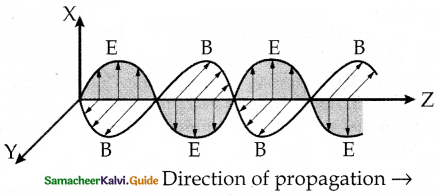Question 6.
Two students A and B prepare the following table about the electromagnetic waves. Rewrite this table in its corrected form:

 Student Direction of Peak value of Electric field Magnetic field Propagation Electric field Magnetic field A Along x-axis Along x-axis Along y-axis E B = cE B Along y-axis Along z-axis Along x-axis E = cB B

Correction for Student ‘A’

 Electric field Magnetic field Peak value of Electric field Magnetic field Along the x-axis or Along the z-axis Along the z-axis or Along the x-axis E B = $$\frac{\mathrm{E}}{\mathrm{C}}$$

No correction for student ‘B’

XII. Conceptual Questions:

Question 1.
Why can light travel in a vacuum, whereas sound cannot do so?

1. Light waves are electromagnetic in nature in which the electric and magnetic field variations do not require a medium for their propagation.
2. Sound waves are mechanical in nature which require an inertial medium, for their propagation.

Question 2.
State the reason why microwaves are best suited for long-distance transmission of signals.

1. Microwaves have wavelengths of the order of a few millimeters. Due to their short wavelengths, these are not diffracted (bent) much by objects of normal dimensions.
2. So they can be used to transmit signals in a particular direction as required in a radar system.

Question 3.
Welders wear special goggles or face masks with glass windows to protect their eyes from electromagnetic radiation. Name the radiations and write the range of their frequency.
To protect their eyes from large amounts of harmful UV radiation produced by the welding arc. The wavelength of UV radiation is from 1 nm to 400 nm.

Question 4.
How do you convince yourself that electromagnetic waves carry energy and momentum?

1. When an electromagnetic wave interacts with matter, its electric and magnetic fields set in oscillation the charges present in the matter.
2. The charges thus acquire energy and momentum from the e.m. wave showing that it carries energy and momentum.
3. When the sun shines on our hands, the energy absorbed from the e.m. waves warms our hands.
4. An e.m. wave carries momentum. When it falls on a surface, it exerts pressure called radiation pressure.

Question 5.
State the condition under which a microwave oven heats up a food item containing water molecules most efficiently.

1. In a microwave oven, the frequency of the microwaves must match the resonant frequency of the water molecules so that energy from the waves is transferred efficiently to the kinetic energy of the molecules.
2. This increases the temperature of the food item sufficiently.Question 6.
It is because the radio waves of short wave band are easily reflected back to the earth by the ionosphere

Question 7.
It is necessary to use satellites for long-distance TV transmission. Why?

1. TV signals being of high frequency are not reflected by the ionosphere.
2. Also, ground wave transmission is possible only upto a limited range.
3. That is why satellites are used for long-distance TV transmission.

Question 8.
Optical and radio telescopes are built on the ground but x-ray astronomy is possible only from satellites orbiting the earth. Why?

1. The earth’s atmosphere is transparent to visible light and radio waves but it absorbs x- rays.
2. X-ray astronomy is possible only from satellites orbiting the earth.
3. These satellites orbit at a height of 36,000 km, where the atmosphere is very thin and x-rays are not absorbed.

Question 9.
The small ozone layer on top of the stratosphere is crucial for human survival. Why?
The ozone layer absorbs ultraviolet radiation from the sun and prevents it from reaching the earth and causing damage to life.

Question 10.
If the earth did not have an atmosphere, would its average surface temperature be higher or lower than what it is now?

1. The earth radiates infrared waves which are reflected by the gases in the lower atmosphere. This phenomenon, called the Greenhouse effect, keeps the earth warm.
2. So if the earth did not have an atmosphere, its average temperature would be low due to the absence of the Greenhouse effect.

Question 11.
Some scientists have predicted that a global nuclear war on the earth would be followed by a severe nuclear winter’ with a devastating effect on life on earth. What might be the basis of this prediction?
The clouds produced by a global nuclear war would perhaps cover substantial parts of the sky preventing solar light from reaching many parts of the globe. This would cause a ‘nuclear winter’.Question 1.
A parallel plate capacitor has circular plates, each of radius 5.0 cm. It is being charged so that the electric field in the gap between its plates rises steadily at the rate of 1012 V m-1 s-1. What is the displacement current?
Solution:
Radius, r = 5cm = 5 x 10-2 m
The rate okf electric frield, $$\frac { dE }{ dt }$$ = 1012 V m-1 s-1
Displacement current, Id = ε0 $$\frac {{ dφ }_{E}}{ dt }$$ =ε0 $$\frac { d }{ dt }$$ (EA) = ε0 (πr2) $$\frac { dE }{ dt }$$
= 8.85 x 10-12 x 3.14 x (5 x 10-2)2 x 1012
Id= 0.069
Id = 0.07 (or) 70 mA

Question 2.
The oscillating magnetic field in a plane electromagnetic wave is given by By = (8 × 10-6) sin [2 × 1011t + 300πx] T
(i) calculate the wavelength of the electromagnetic wave.
(ii) Write down the expression for the oscillating electric field.
Given By = (8 × 10-6) sin [2 × 1011t + 300πx] T
Standard equation is By = B0 sin (sin 2π $$\left(\frac{x}{\lambda}+\frac{t}{T}\right)$$]
on comparing, $$\frac{2 \pi}{\lambda}$$ = 300π and B0 = 8 × 10-6 T

(i) wavelength, λ = $$\frac{2 \pi}{300 \pi}=\frac{1}{150}$$ m = 0.67 cm.

(ii) E0 = cB0 = 3 × 108 ×8 × 10-6 = 2400 Vm-1
The electric field is perpendicular to the direction of propagation (x-axis) and the direction of magnetic field (y-axis). Hence the expression for the oscillating electric field is
Ez = 2400 sin [2 × 1011t + 300πx] Vm-1

Question 3.
The oscillating electric field of an electromagnetic wave is given by:
Ey = 30 sin [2 × 1011t + 300πx] Vm-1
(a) Obtain the value of the wavelength of the electromagnetic wave.
(b) Write down the expression for the oscillating magnetic field.
Given data:
Ey = 30 sin [2 × 1011t + 300πx] Vm-1
Standard equation is Ey = E0 sin [2π$$\left(\frac{x}{\lambda}+\frac{t}{T}\right)$$]
on comparing, $$\frac{2 \pi}{\lambda}$$ = 300π and E0 = 30 Vm-1

(i) wavelength, $$\frac{2 \pi}{\lambda}$$ = 300π = $$\frac{1}{150}$$ m = 0.67 cm

(ii) B0 = $$\frac{E_{0}}{c}=\frac{30}{3 \times 10^{8}}$$ = 10-7 T

The magnetic field is perpendicular to the direction of propagation (x-axis) and the direction of the electric field (y-axis). So the expression for the magnetic field is
Bz = 10-7 sin [2 × 1011t + 300πx] T

Question 4.
Radiation of energy E falls normally on a perfectly reflecting surface. Find the momentum transferred to the surface.
Solution:
Momentum of radiation of energy E is P = $$\frac { E }{ C }$$
Since the radiation is completely reflected, its momentum changes by $$\frac { 2E }{ C }$$
Therefore, by the law of conservation of momentum the momentum transferred to the surface is $$\frac { 2E }{ C }$$.Question 5.
A charged particle oscillates about its mean equilibrium position with a frequency of 109 Hz. What is the frequency of the electromagnetic waves produced by the oscillator?
According to Maxwell, a charged particle oscillating with a frequency of 109 Hz, produces electromagnetic waves of the same frequency 109 Hz.

Question 6.
The amplitude of the magnetic field part of a harmonic electromagnetic wave in a vacuum is B0 = 510 nT. What is the amplitude of the electric field part of the wave?
Here B0 = 510 nT = 510 × 10-9 T
Amplitude of the electric field, E0 = cB0
= 3 × 108 × 510 × 10-9
= 153 NC-1.

Question 7.
Suppose that the electric field amplitude of an electromagnetic wave is E0 = 120 NC-1 and that its frequency is v = 50.0 MHz.
(a) Determine, B0, ω, k and λ,
(b) Find expressions for $$\overrightarrow{\vec{E}}$$ and $$\vec{B}$$
Here E0 = 120 NC-1
v = 50.0 MHz = 50 × 106 Hz

a) B0 = $$\frac{E_{0}}{c}=\frac{120 \mathrm{NC}^{-1}}{3 \times 10^{8} \mathrm{~ms}^{-1}}$$ = 4 × 107 T

ω = 2πv = 2 × 3.14 × 50 × 106 = 3014 × 108 rad s-1

$$\kappa=\frac{\omega}{c}=\frac{3.14 \times 10^{8}}{3 \times 10^{8}}=$$ = 1.05 ms-1

λ = $$\frac{c}{v}=\frac{3 \times 10^{8}}{50 \times 10^{6}}$$ = 6.00 m

(b) If the wave is propagating along x-axis, then field $$\overrightarrow{\vec{E}}$$ will be along y-axis and field $$\vec{B}$$ along z-axis.
E0 = 120 sin (1.05x – 3.14 × 108t) ĵ NC-1
Where x is in metre and t in second.
B = B0 sin (kx – ωt) k̂.
= 4 × 10-7 sin (1.05x – 3.14 × 108t) ĵ tesla.

Question 8.
About 5% of the power of a 100 W light bulb is converted to visible radiation. What is the average intensity of visible radiation:
a) at a distance of 1 m from the bulb?
b) at a distance of 10 m
The bulb, as a point source, radiates light in all directions. At a distance of r m, the surface area of the surrounding sphere,
A = 4πr²
∴ Average Intensity = $$\frac{\text { Energy / time }}{\text { Area }}=\frac{\text { Power }}{\text { Area }}=\frac{\text { Power }}{4 \pi \mathrm{r}^{2}}$$

(a) Average intensity of visible radiation at a distance of 1 m = $$\frac{5 \% \text { of } 100 \mathrm{~W}}{4 \pi(1 \mathrm{~m})^{2}}$$ = 0.4 Wm-2

(b) Average intensity of visible radiation at a distance of 10 m = $$\frac{5 \% \text { of } 100 \mathrm{~W}}{4 \pi(10 \mathrm{~m})^{2}}$$ = 0.004 Wm-2XIV. Five Marks:

Question 1.
Considering the case of a parallel plate capacitor being charged, show how one is required to generalize Ampere’s circuital law to include the term due to displacement current.
1. According to Amperes circuited law,

$$\oint_{\mathrm{C}} \overrightarrow{\mathrm{B}} \cdot \overrightarrow{\mathrm{d} l}=\mu_{0} \mathrm{I}$$ ………..(1)
2. As the current I flows across the area bounded by loop C1, so

$$\oint_{\mathrm{C}_{1}} \overrightarrow{\mathrm{B}} \cdot \overrightarrow{\mathrm{d} l}=\mu_{0} \mathrm{I}$$ ………(2)
3. But the area bounded by C2 lies in the region between the capacitor plates, so no current flows across it.

$$\oint_{\mathrm{C}_{2}} \overrightarrow{\mathrm{B}} \cdot \overrightarrow{\mathrm{d}}=0$$ ………..(3)
4. Imagine the loops C1 and C2 to he infinitesimally close to each othcr. Then we must have

$$\oint_{\mathrm{C}_{1}} \overrightarrow{\mathrm{B}} \cdot \overrightarrow{\mathrm{d} l}=\oint_{\mathrm{C}_{2}} \overrightarrow{\mathrm{B}} \cdot \overrightarrow{\mathrm{d} l}$$ …………(4)

This result is inconsistent with the eqns. (2) and (3). To remove this inconsistency, Maxwell argued that a changing electric field (during charging) between the capacitor plates must induce a magnetic field which, in turn,
must be associated with a current. Maxwell called this current as the displacement current. It is given by
ID = ε0 $$\frac{d \phi_{E}}{d t}$$
The total current must be,
I = Iconduction + Idisplacement = IC ε0 $$\frac{d \phi_{E}}{d t}$$
Hence, the generalised form of the Ampere’s circuit law is
$$\oint_{\mathrm{B}} \overrightarrow{\mathrm{d} l}=\mu_{0} \mathrm{I}\left[I_{\mathrm{C}}+\varepsilon_{0} \frac{d \phi_{E}}{d t}\right]$$

Question 2.
Show that during the charging of a parallel plate capacitor, the rate of change of charge on each plate equals ε0 times the rate of change of electric flux (φE) linked with it. what is the name given to the term ε0 $$\frac{d \phi_{E}}{d t}$$?
If A be the area of the capacitor plates and q be the charge on the plates at any instant t during the charging process, then the electric field in the gap will beThe term ε0 $$\frac{d \phi_{E}}{d t}$$ is called displacement vector.Question 3.
Consider a plane em. a wave traveling with speed c in the positive z-direction.
(i) Use Faraday’s law to show the E = cB
(ii) Use modified Ampere’s circuital law to show that $$c=\frac{1}{\sqrt{\mu_{0} \varepsilon_{0}}}$$.
Let $$\vec{E}$$ be in the x-direction and B in the y-direction.
(i) consider a rectangular loop in the x-z plane with one side of length 1 parallel to E Suppose at any instant, the rectangle is partially on the left of the wavefront and partially on the right of the wavefront.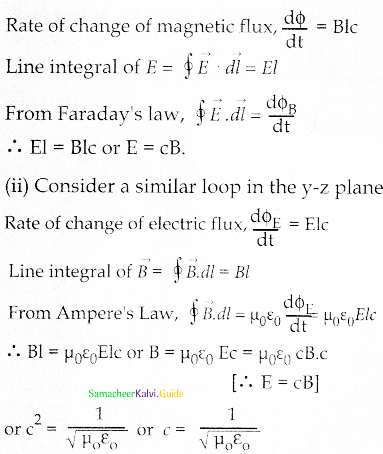Question 4.
A capacitor, made of two parallel plates each of plate area A and separation d, is being charged by an external ac source. Show that the displacement current inside the capacitor is the same as the current charging the capacitor.
E = $$\frac{\sigma}{\varepsilon_{0}}=\frac{q}{\varepsilon_{0} A}$$
$$\phi_{E}=E A=\frac{q}{\varepsilon_{0} A} \cdot A \frac{q}{\varepsilon_{0}}$$
ID = $$\varepsilon_{0} \frac{\mathrm{d} \phi}{\mathrm{dt}}=\varepsilon_{0} \frac{\mathrm{d}}{\mathrm{dt}}\left[\frac{\mathrm{q}}{\varepsilon_{0}}\right]=\frac{\mathrm{dq}}{\mathrm{dt}}$$
But $$\frac{\mathrm{dq}}{\mathrm{dt}}$$ = rate at which charge flows to positive plate through the conducting wire.